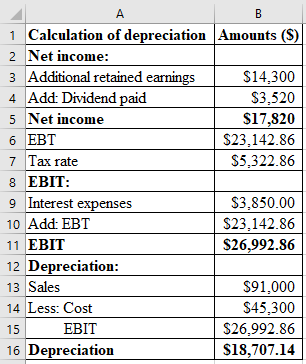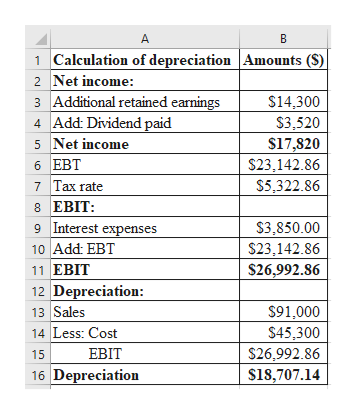Given the following information for O'Hara Marine Co., calculate the depreciation expense: sales = \$91,000; costs = \$45,300; addition to retained earnings = \$14,300; dividends paid = \$3,520; interest expense = \$3,850; tax rate = 23 percent

Question

Given the following information for O'Hara Marine Co., calculate the depreciation expense: sales = \$91,000; costs = \$45,300; addition to retained earnings = \$14,300; dividends paid = \$3,520; interest expense = \$3,850; tax rate = 23 percent

Step 1

Calculation of depreciation expenses:

Depreciation expenses is \$18,707.14.Excel spread sheet:

...help_outlineImage TranscriptioncloseA B 1 Calculation of depreciation Amounts (S) Net income: 3 Additional retained earnings 4 Add: Dividend paid 5 Net income 6 EBT 7 Tax rate 8 EBIT 9 Interest expenses 10 Add: EBT 11 ЕBIT \$14,300 \$3,520 \$17,820 \$23,142.86 \$5,322.86 S3,850.00 \$23,142.86 \$26,992.86 12 Depreciation: 13 Sales 14 Less: Cost \$91,000 \$45,300 S26,992.86 ЕBIT 15 16 Depreciation \$18,707.14 N fullscreen

Want to see the full answer?

See Solution

Want to see this answer and more?

Our solutions are written by experts, many with advanced degrees, and available 24/7

See Solution
Tagged in